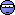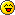Gravity

::  ::  ::Gravity

Create a program that finds the gravitational force acted upon between two given masses and a given distance.

AND I POSTED THIS IN THE C++ FORUM FOR A REASONLast edited by Admin on Wed Dec 23, 2009 6:29 am; edited 1 time in totalPirate King

Posts : 559Re: Gravity

now i tried this myself. and i got the thing written up but i keep getting a damn error. so ....code is in spoiler

Spoiler:
Code:
`#include <iostream>#include <cmath>using namespace std;double compute(double m1, double m2, double d){            static double G =6.67428*(double pow(10,-11));      double force=((G*m1*m2)/(double pow(d,2)));/*you can just return this thing instead of       declaring a whole new variable for this line,        but this is just for making the code more legible.*/      return force;}      int main(){    double mass1,mass2;    double distance;      cout<<"This is the gravitational force calculating program. All input must be in SI units!\n";  cout<<"Please input two masses in the following lines. Remember they must be in kilograms.\n\n";    cout<<"First Mass: ";  cin>>mass1;  cout<<"\nSecond Mass: ";  cin>>mass2;    cout<<"\n\nOK so far so good, now we need the distance between the centers of the two masses."<<endl;  cout<<"\nDistance: ";  cin>>distance;    cout<<"Time to work some programming magic here..... answer: "<<compute(mass1,mass2,distance);  cin.get();  return 0;}`

_________________Pirate King

Posts : 559Re: Gravity

hmm i got an interesting idea. if i don't use the math library it compiles fine. wtf?

well here it is

edit: wait this doesn't work yet....gimme a sec...

edit: ok after tweaking around i found that if you enter a double into the DISTANCE variable, it screws up the program. .... wtf.?
Spoiler:
Code:
`#include <iostream>using namespace std;double compute(double m1, double m2, double d){            static double G =.0000000000667428;      double force=((G*m1*m2)/d*d);/*you can just return this thing instead of       declaring a whole new variable for this line,        but this is just for making the code more legible.*/      return force;}      int main(){    double mass1,mass2;    double distance;      cout<<"This is the gravitational force calculating program. All input must be in SI units!\n";  cout<<"Please input two masses in the following lines. Remember they must be in kilograms.\n\n";    cout<<"First Mass: ";  cin>>mass1;  cout<<"\nSecond Mass: ";  cin>>mass2;    cout<<"\n\nOK so far so good, now we need the distance between the centers of the two masses."<<endl;  cout<<"\nDistance: ";  cin>>distance;    cout<<"Time to work some programming magic here..... answer: "<<compute(mass1,mass2,distance);  cin.get();  return 0;}`Pirate King

Posts : 559Re: Gravity

Code:
`double compute(double m1, double m2, double d){          static double G =.0000000000667428;      double force=( G*( (m1*m2)/(d*d) ) );      return force;}`

try this. . .

I'm not checking the code, I'm just correcting one BLATANT errorPaul
Pickaxe

Posts : 611Re: Gravity

when i enter a double for the value of distance, the console just disappearsi've had this problem before your correction and still afterwards.

_________________Pirate King

Posts : 559Re: Gravity

Code:
`double compute(double m1, double m2, double d){      static double G =0.0000000000667428;      double force=( G*( (m1*m2)/(d*d) ) );      return force;}`Paul
Pickaxe

Posts : 611Re: Gravity

i love how you guys just post code willy-nilly without explanationsLunsfordium
[Blankie]

Posts : 194Re: Gravity